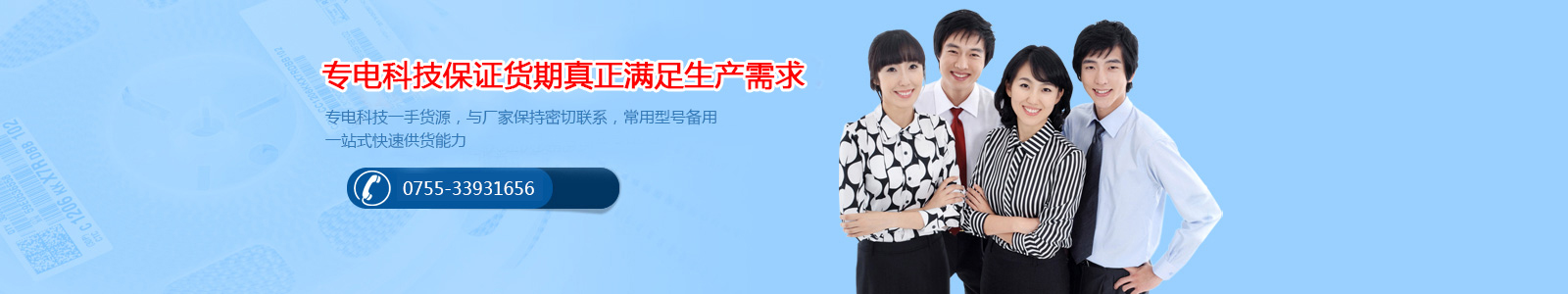# 如何通过封装料盘识别三星贴片电容的耐压值

### 发布日期：2017-11-07 作者：zhu 点击：

CL  03  B  104  K   Q  8  N  N   N   C

1   2   3   4   5   6  7  8  9  10  11

1 系列编码：

CL=积层陶瓷电容

2 尺寸编码

03=0201(0603) 21=0805(2012) 42=1808(4520)

05=0402(1005) 31=1206(3216) 43=1812(4532)

10=0603(1608) 32=1210(3225) 55=2220(5750)

14=0504(1410) 01=0306(0816) 12=0508(1220)

3 介质：

I类 II类

C=C0G S=S2H L=S2L

P=P2H T=T2H

R=R2H U=U2J

A=X5R F=Y5V

B=X7R X=X6S

4 容量

5 电容的误差：

B=±0.1pf F=±1pf±1% K=±10%

C=±0.25pf G=±2% M=±20%

D=±0.5pf? J=±5% Z=+80/-20%

6 额定电压：

R=4V O =16V B =50V E = 250V I = 1000V

Q=6.3V A =25V C=100V G = 500V J = 2000V

P =10V L =35V D =200V H = 630V K= 3000V

7 厚度：

3=0.30毫米 A=0.65毫米 M=1.15毫米 I=2.00毫米 Q=1.25毫米

5=0.50毫米 C=0.85毫米 F=1.25毫米 J=2.50毫米 V=2.50毫米

8=0.80毫米 D=1.00毫米 H=1.60毫米 L=3.20毫米

8 内电极

A=常规产品 钯/银/镍屏蔽/锡 100%

N=常规产品 镍/铜/镍屏蔽/锡 100%

G=常规产品 铜/铜/镍屏蔽/锡 100%

L=低侧面产品 镍/铜/镍屏蔽/锡 100%

9 产品编码

A =阵列(2-元素)  L =LICC

B =阵列(4-元素)  N =常规

P =自动          C=高频

10 特殊编码

11 包装编码

B=散装 O=纸版箱料带,10英寸料盘 E=压花纸版箱,7英寸料盘

P=散装箱 D=纸版箱料带,13英寸料盘(10000ea) F=压花纸版箱,13英寸料盘

C=纸版箱料带,7英寸料盘 L=纸版箱料带,13英寸料盘(15,000ea) S=压花纸版箱,10英寸料盘

### 相关标签：三星贴片电容

#### 相关新闻：